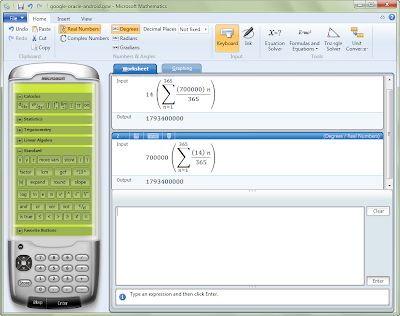X
Innovation

# How much money Google really makes from Android

A lot of blogs are suggesting that Google's earnings from Android are coming in at a spectacular \$3.65 billion dollars a year.

A lot of blogs are suggesting that Google's earnings from Android are coming in at a spectacular \$3.65 billion dollars a year. It's a big number, but sadly erroneous.

If you do a quick back of an envelope calculation, based on numbers from Oracle's submissions to the court case where it's suing Google over its alleged unlicensed use of Java, the current Android activation rate of 700,000 devices a day and a per device average revenue of \$14 per year gives you a figure of around \$10 million dollars per year per day in earnings from those devices. A lot of people have been simply multiplying that by 365 to get an approximate annual run rate for Google's earnings.

Sadly there's a logical error in that sum, as it assumes that Google earns the same amount in one year from a device sold on the 31st of December as for one sold on the 1st of January. That's clearly not the case, so we need to consider the run rate as a sum of a simple series – one that calculates the annual number of device activations and uses that to give us an overall annual run rate. A quick estimate is that the value of Android devices to Google (and hence to Oracle!) should be about half the value most people are suggesting.

The equation we want is a lot more like this:Using that equation, the numbers we'll get from that calculation turn out to be as expected, about half of what that the back of the envelope calculation comes up with – a run rate for Android of around \$1.79 billion a year. Yes, it's still a lot of money, but it’s nowhere the number that people are suggesting.

Of course it's not going to be completely accurate – for one thing the rate of Android activations isn't constant, and we're assuming a zero starting point (something that's obviously not true). It would be interesting to see Google's actual numbers, but that's going to have to wait until they get their day in court.

So remember folks, it's always a good idea to do the maths – but it's an even better idea to formulate the problem correctly. That way you're much more likely to get something close to the real answer.

It's a problem that's also given me a chance to play with a rather useful piece of free software from Microsoft, Microsoft Mathematics. It's a very effective graphing scientific calculator, with lots of useful features – including a set of calculus tools, which handle equation formatting and display.Simon Bisson

Editorial standards
ZDNET